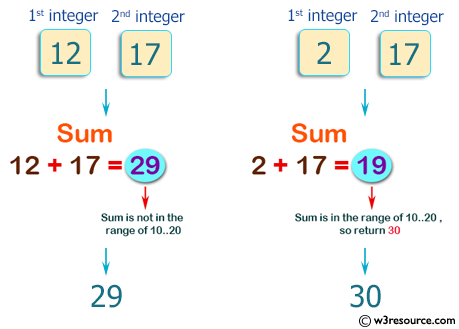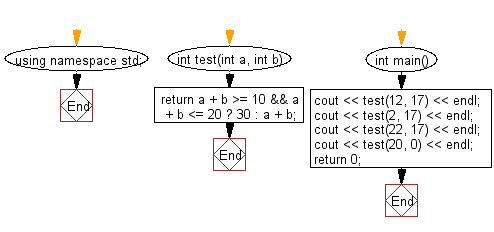﻿ C++ : Sum of the two integers in the range 10..20# C++ Exercises: Compute the sum of the two given integers, if the sum is in the range 10..20 inclusive return 30

## C++ Basic Algorithm: Exercise-37 with Solution

Write a C++ program to compute the sum of the two given integers. If the sum is in the range 10..20 inclusive return 30.

Sample Solution:

C++ Code :

``````#include <iostream>

using namespace std;

int test(int a, int b)
{
return a + b >= 10 && a + b <= 20 ? 30 : a + b;
}

int main()
{
cout << test(12, 17) << endl;
cout << test(2, 17) << endl;
cout << test(22, 17) << endl;
cout << test(20, 0) << endl;
return 0;

}
``````

Sample Output:

```29
30
39
30
```

Pictorial Presentation:Flowchart:C++ Code Editor: